# Quantitative Analysis for Managerial Decision Making

### Course description

Quantitative analysis for managerial decision making has other names like management science and operations research. Thus, it is applied to problems that concern how to conduct and coordinate the operations/ the activities within an organization. Operations research has been applied extensively in activities in such diverse areas as manufacturing, transportation, construction, telecommunications, financial planning, health care, the military, and public services. Therefore, the breadth of application is unusually wide. Operations research involves research on operations. The research part of the name means that operations research uses an approach that resembles the way research is conducted in established scientific fields. The scientific method is used to investigate the problem of concern. The term management science sometimes is used as a synonym for operations research. In particular, the process begins by carefully observing and formulating the problem, including gathering all relevant data. The next step is to construct a scientific, typically mathematical, model that attempts to abstract the essence of the real problem. It is then hypothesized that this model is a sufficiently precise representation of the essential features of the situation that the conclusions/solutions obtained from the model are also valid for the real problem. Suitable experiments are conducted to test this hypothesis, modify it as needed, and eventually verify some form of the hypothesis. Specifically, operation research is also concerned with the practical management of the organization. Therefore, to be successful, operation research must also provide positive, understandable conclusions to the decision maker/s when they are needed.

### Course outcomes

Upon the completion of this course, students will be able to:

• Understand the essence of management science/quantitative analysis/operation research
• Formulate a real-world problem as a mathematical programming model and solve the model
• Build and solve transportation models and assignment models
• Solve network models such as the shortest path, minimum spanning tree, and maximum flow problems
• Understand how to model and solve problems related to inventory management
• Model a dynamic system as a queuing model and compute important performance measures
• Understand the characteristics of different types of decision-making environments and the appropriate decision making approaches and tools to be used in each type

### Course contents

Click the down arrow icon [ 🔽 ] to expand and collapse the course topics.

🔽 0 h 58 min | Introduction
• Problem solving and decision making
• Quantitative analysis and decision making
• Quantitative analysis
• Models of cost, revenue, profit and breakeven analysis
• Management science techniques
🔽 4 h 35 min | Linear Programing
• Introduction to linear programming
• Problem formulation
• Linear programming applications
• Maximization problem
• Minimization problem
• Methods to solve linear programming
• Graphical solution procedure
• Simplex method
• Special cases
• Linear programming: Sensitivity analysis and interpretation of solution
🔽 2 h 25 min | Transportation and Assignment Problem – A Special Case of Linear Programing
• Transportation problem
• Methods of solving transportation problem
• Assignment problem
• Methods of solving assignment problem
• Shortest-route problem
• Maximal flow problem
🔽 1 h 33 min | Network Model
• Network conventions
• Network diagram
• Critical path analysis
• PERT networks
• Reducing project completion time
• Crashing
🔽 1 h 00 min | Decision Analysis
• Decision making environments
• Decision making without probabilities
• Decision making with probabilities
🔽 0 h 47 min | Game Theory
• The concepts of game theory and its basic elements
• The formulation of two-person, zero-sum games
• Characteristics and principles of a game

### This course includes: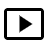11 h 18 min recorded video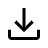Downloadable resources (books and articles)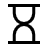One year access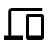Access on mobile and TV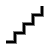Advanced Level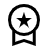Certificate of completion

#### Self-paced

7,900 Br
1 year of access
This course does not have any sections.